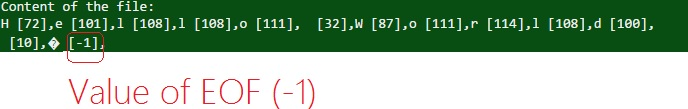# Value of EOF in c programming language.

EOF is a predefined MACRO with the value of -1 that means EOF is not a character. So EOF is returned through the function which is going to read content from the file.

Here is a C program, that will print the value of EOF

```#include <stdio.h>

int main(){

printf("Value of \"EOF\" is = %d\n",EOF);
return 0;
}
```

Output

```Value of "EOF" is = -1
```

Another program, to read and print characters (integer values) from file

There is a file "text1.txt" containing "Hello World"

```#include <stdio.h>

int main(){
FILE *fp;
int ch;

fp=fopen("text1.txt","r");
if(fp==NULL){
printf("Error in file opening...\n");
return -1;
}

printf("Content of the file:\n");
/*here we are using infinite loop to print value of
EOF, loop will be terminate as we got EOF*/
while(1){
printf("%c [%d],",ch,ch);
if(ch==EOF){
break; //terminate loop
}
}

//close the file
fclose(fp);

return 0;
}
```

Output

```H ,e ,l ,l ,o , ,W ,o ,r ,l ,d ,
,� [-1],
```